# Methods and formulas for benchmark Zs for overall capability in Normal Capability Analysis for Multiple Variables

## Z.LSL, Z.USL, and Z.Bench for overall capability

Benchmark Z statistics for overall capability are calculated by finding the Z value using the standard normal (0,1) distribution for the corresponding statistics.

where:

### Notation

TermDescription
Φ (X) Cumulative distribution function (CDF) of a standard normal distribution
Φ-1 (X)Inverse CDF of a standard normal distribution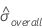Overall standard deviation

## Confidence intervals for Z.bench for a process with two specification limits

### Two-sided interval

where

To calculate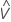, substitute the sample estimates for the parameters in the formula for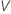:

where

### One-sided upper confidence bound

To calculate a one-sided upper confidence bound, change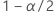to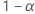in the definition of U.

### Notation

TermDescription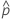the estimated tail probabilities outside of the specificataion limits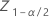the (1 - α / 2)th percentile of the standard normal distribution
αthe alpha for the confidence levelthe process mean (estimated from the sample date or a historical value)
sthe sample standard deviation within subgroups
υthe degrees of freedom for s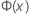the Cumulative Distribution Function (CDF) from a standard normal distribution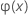the Probability Density Function (PDF) from a standard normal distribution
USLthe upper specification limit
LSLthe lower specification limit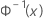the inverse CDF from a standard normal distribution

## Confidence intervals for Z.bench for a process with one specification limit

The calculations for the confidence interval for Z.Bench depend on which specification limit the process has.

### Lower specification limit, one-sided confidence bound

Minitab solves the following equation to find p1:

where

### Upper specification limit, one-sided confidence bound

Minitab solves the following equation to find p2:

where

### Notation

TermDescription
LSLthe lower specification limit
USLthe upper specification limit
αthe alpha for the confidence levelthe Cumulative Distribution Function (CDF) from a standard normal distributionthe inverse CDF from a standard normal distributionthe (1 - α/2)th percentile of the standard normal distribution
Nthe total number of measurements
υthe degrees of freedom for sthe process mean (estimated from the sample date or a historical value)
sthe sample overall standard deviation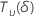a random variable that is distributed as a non-central t distribution with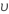degrees of freedom and non-centrality parameter δ